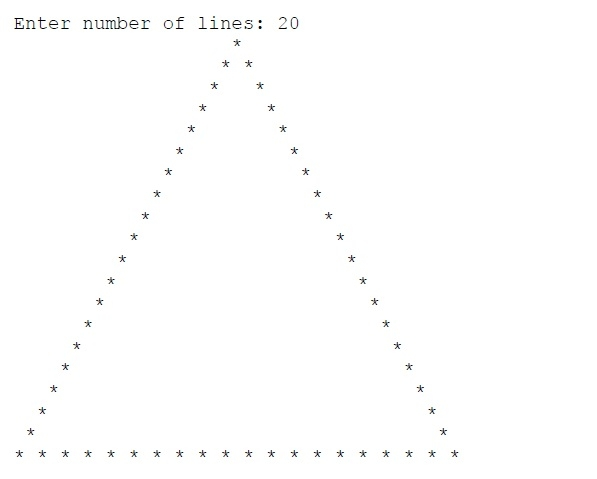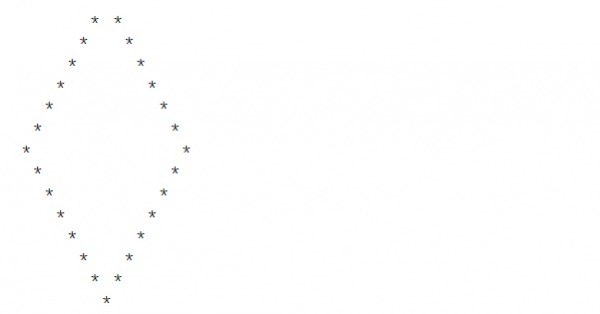# Program to print hollow pyramid and diamond pattern in C++

Here we will see how to generate hollow pyramid and diamond patterns using C++. We can generate solid Pyramid patterns very easily. To make it hollow, we have to add some few tricks.

## Hollow Pyramid

For the pyramid at the first line it will print one star, and at the last line it will print n number of stars. For other lines it will print exactly two stars at the start and end of the line, and there will be some blank spaces between these two starts.

## Example Code

#include <iostream>
using namespace std;
int main() {
int n, i, j;
cout << "Enter number of lines: ";
cin >> n;
for(i = 1; i<=n; i++) {
for(j = 1; j<=(n-i); j++) { //print the blank spaces before star
cout << " ";
}
if(i == 1 || i == n) { //for the first and last line, print the stars continuously
for(j = 1; j<=i; j++) {
cout << "* ";
}
}else{
cout << "*"; //in each line star at start and end position
for(j = 1; j<=2*i-3; j++) { //print space to make hollow
cout << " ";
}
cout << "*";
}
cout << endl;
}
}

## Output## Hollow Diamond

For the diamond at the first line and at the last line it will print one star. For other lines it will print exactly two stars at the start and end of the line, and there will be some blank spaces between these two starts. Diamond has two parts. The upper half and the lower half. At the upper half we have to increase the space count, and for the lower half, we have to decrease the space count. Here the line numbers can be divided into two parts by using another variable called mid.

## Example Code

#include <iostream>
using namespace std;
int main() {
int n, i, j, mid;
cout << "Enter number of lines: ";
cin >> n;
if(n %2 == 1) { //when n is odd, increase it by 1 to make it even
n++;
}
mid = (n/2);
for(i = 1; i<= mid; i++) {
for(j = 1; j<=(mid-i); j++) { //print the blank spaces before star
cout << " ";
}
if(i == 1) {
cout << "*";
}else{
cout << "*"; //in each line star at start and end position
for(j = 1; j<=2*i-3; j++) { //print space to make hollow
cout << " ";
}
cout << "*";
}
cout << endl;
}
for(i = mid+1; i<n; i++) {
for(j = 1; j<=i-mid; j++) { //print the blank spaces before star
cout << " ";
}
if(i == n-1) {
cout << "*";
}else{
cout << "*"; //in each line star at start and end position
for(j = 1; j<=2*(n - i)-3; j++) { //print space to make hollow
cout << " ";
}
cout << "*";
}
cout << endl;
}
}

## Output